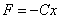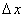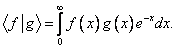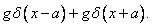# Physics 751 Midterm I

October 5, 2007

1. Suppose an electron is attracted towards the origin by a force, where C is a positive constant, r is distance from the origin.

(a) What is the radius of the smallest circular Bohr orbit?

(b) What is the energy in the nth circular Bohr orbit?

(c) Now suppose the electron oscillated on a line through the origin, so.  Use the Uncertainty Principle to estimate the linear spreadof the wave function.

2. (a) Suppose the inner product for real functions defined forisBegin with the set 1, x, x2, … and construct the first three elements of an orthonormal basis.

(b)  Find the eigenvalues and the eigenvectors of:3.  Consider the one-dimensional Schrodinger equation for an electron of mass m in a potentialFor what values of g is there a bound state with extremely weak binding energy ε?

(Hint: there are two values, one of which is extremely small, the other isn’t.)